# JP2009520306A - Parallel multirate circuit simulation - Google Patents

## Info

Publication number
JP2009520306A
JP2009520306A JP2008547473A JP2008547473A JP2009520306A JP 2009520306 A JP2009520306 A JP 2009520306A JP 2008547473 A JP2008547473 A JP 2008547473A JP 2008547473 A JP2008547473 A JP 2008547473A JP 2009520306 A JP2009520306 A JP 2009520306A
Authority
JP
Japan
Prior art keywords
matrix
circuit
method
system
preconditioned
Prior art date
Legal status (The legal status is an assumption and is not a legal conclusion. Google has not performed a legal analysis and makes no representation as to the accuracy of the status listed.)
Granted
Application number
JP2008547473A
Other languages
Japanese (ja)
Other versions
JP4790816B2 (en
Inventor
ヤン、バオリン
Original Assignee
ジェミニ デザイン テクノロジー、インコーポレーテッド
Priority date (The priority date is an assumption and is not a legal conclusion. Google has not performed a legal analysis and makes no representation as to the accuracy of the date listed.)
Filing date
Publication date
Priority to US75221705P priority Critical
Priority to US60/752,217 priority
Priority to US78937606P priority
Priority to US60/789,376 priority
Application filed by ジェミニ デザイン テクノロジー、インコーポレーテッド filed Critical ジェミニ デザイン テクノロジー、インコーポレーテッド
Priority to PCT/US2006/048551 priority patent/WO2007075757A2/en
Publication of JP2009520306A publication Critical patent/JP2009520306A/en
Application granted granted Critical
Publication of JP4790816B2 publication Critical patent/JP4790816B2/en
Application status is Active legal-status Critical
Anticipated expiration legal-status Critical

## Images

•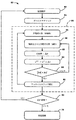•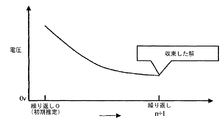•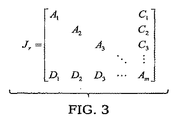•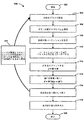•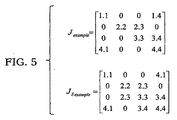•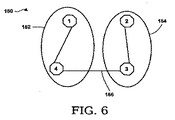•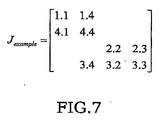•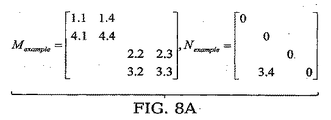•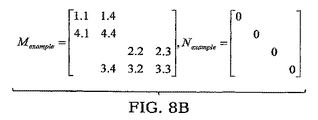•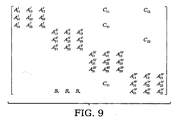•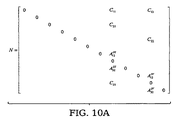•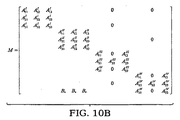•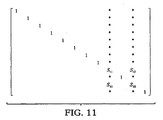•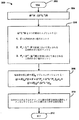•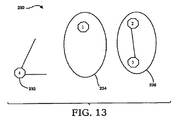•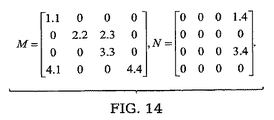•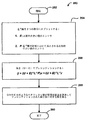•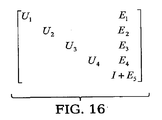## Classifications

• GPHYSICS
• G06COMPUTING; CALCULATING; COUNTING
• G06FELECTRIC DIGITAL DATA PROCESSING
• G06F17/00Digital computing or data processing equipment or methods, specially adapted for specific functions
• G06F17/50Computer-aided design
• G06F17/5009Computer-aided design using simulation
• G06F17/5036Computer-aided design using simulation for analog modelling, e.g. for circuits, spice programme, direct methods, relaxation methods

## Abstract

A computer implemented method for solving parallel equations in circuit simulation is described. The method includes dividing the circuit Jacobian matrix into loosely coupled partitions, reordering the voltage vector and matrix according to the partition, and splitting the Jacobian matrix into two matrices M and N, Is a matrix suitable for parallel processing, and N is a coupling matrix. M and N are preconditioned to form M −1 Jx = (I + M −1 N) x = M −1 r, and the Jacobian matrix J is solved using an iterative solution.
[Selection] Figure 4

## Description

In Electronic Design Automation (EDA), software has been developed that can be used by integrated circuit designers to explore and verify their designs. As the semiconductor industry has entered the nanometer era, designers need to use EDA tools to determine if the circuits being manufactured have nanometer-level effects. If the design was not verified, the integrated circuit produced would malfunction with a high probability and would have to be redesigned and expensive.

Circuit simulation is the most accurate way to verify whether a circuit design works. FIG. 1 is a flow diagram 50 illustrating a typical transformation that occurs in manufacturing a circuit from when the initial design is realized. Block 52 shows the initial circuit design. This design is broken down into a series of connected device elements 54. Each device element in the design is modeled with an accurate analytical model that has been verified by semiconductor manufacturing factories and manufacturers. If a device element represented by an analytical model is used, a circuit simulator indicated by a block 55 indicated by a broken line can simulate a voltage value and a current value over a certain period. The circuit simulator 55 includes a computer system programmed to perform circuit simulation operations on data. In the circuit simulation, voltage values and current values of all nodes of the circuit are obtained by solving a system (system) of differential algebraic equations (DAEs) represented in the block 56 of the flow diagram 50.

DAE can be discretized using a finite difference method, and an equation is solved by an iterative process using a nonlinear iterative method such as Newton-Raphson method. At each iteration, the nonlinear equations are linearized around the previously obtained solution to produce a linearized system of equations, indicated by block 58. And it is necessary to solve this linear system.

Matrix solving techniques are widely used in many scientific and engineering fields. In EDA, matrix solution technology is indispensable for solving linear equation systems in fields such as circuit simulation.

The circuit equation takes the form:

Where v is a vector of voltages at all nodes simulated by the circuit. Q (v) is the charge at these nodes. i (v) is the current at these nodes. u 0 is the power supply of the circuit.

When solving the above equation, the finite differentiation method is first used to approximate the differential operator. Here, for the sake of explanation, the backward Euler method will be described, but if other finite differentiation methods are used, other methods can be applied as well. The discrete equation system has the form

Here, it is assumed that the time step t m is known, and a solution at the time t m + 1 is obtained. Newton-Raphson iteration can be used to solve nonlinear discrete equations. In an iterative process, an initial estimate v 0 is given. Thereafter, a sequence of solutions v 1 , v 2 ,... Is obtained and converges to a solution of a nonlinear equation as shown in the graph of FIG. In each iteration, the nonlinear equations are linearized around the known solutions v n obtained in the previous iteration. Then, Δv is obtained by solving the following linear equation system.

here

After obtaining Δv, an updated solution v n + 1 = v n + Δv is obtained. This process continues until the following conditions are met:

Here, tol is a small error tolerance. When the condition of Equation 4 is satisfied, the solution is considered to have converged as shown in FIG. 2, and the solution at t m + 1 is as follows.

In conventional Spice circuit simulation, error checking is performed for all nodes, and | Δv | is the maximum absolute value of all entries in the vector Δv, ie, the change in solution at all nodes. When equation 4 is satisfied, the procedure advances one time step and returns to block 56 of flowchart 50 to solve the next system of nonlinear equations corresponding to the next time. In this way, transient fluctuations can be simulated in the modeled circuit.

The voltage value generated at each time is analyzed to determine whether the circuit simulation is consistent with the expected operation of the circuit. For example, the voltage value is compared with a logical analysis of the circuit design. In another example, voltage and current values can be used to perform power and timing analysis of the circuit design. In operation 68, if the simulation does not match the expected operation, the design is changed and the defect is corrected. The procedure then returns to operation 52 under the new circuit design. However, if the voltage is comparable to the expected operation, the circuit design is manufactured as a prototype. That is, the manufacturing circuit 70 is generated.

The main part of solving the linear system is to solve the circuit Jacob matrix, which includes the LU decomposition step and the forward and backward substitution steps in the conventional direct approach. Fast and accurate matrix solving is very important in fast and accurate circuit simulation.

Matrix solving techniques can be divided into two categories. One category is called direct matrix solving. The other category is called iterative matrix solution.

Consider the following linear system in circuit simulation.

Here, J is a Jacobian matrix of the circuit, r is a residual vector, and Δv is an update vector of the node voltage solution.

The direct matrix solving method first LU decomposes matrix J

Here, L is a lower triangular matrix and U is an upper triangular matrix. Thereafter, the following equation is solved to obtain a solution Δv for the linear system.

And

In the following description, for convenience, x is used to represent Δv.

The iterative matrix method tries to obtain or approach a solution while iterating. Under the initial estimate x 0 , the iterative method approaches the solution x in the next process.

Here, n ≧ 0 and B is a preconditioned matrix for iterative solution. For iterative solution to be efficient, this process must be one that can approach the solution with an accuracy acceptable by relatively small number of iterations, the calculation of Bx n must be fast . The Krylov subspace iteration method has sufficient convergence properties and can approach the circuit solution at a reasonable speed. The purpose of preconditioning is to bring matrix B closer to the identity matrix in order to speed up the iterative process.

In circuit simulation, the size of a linear system may become very large, and the pattern of matrix elements may become sparse. For this reason, a standard circuit simulator employs a sparse matrix representation of the matrix A. In a sparse representation of a matrix, it is common to preserve only non-zero matrix elements. A non-zero pattern of a sparse matrix is important for improving the efficiency of LU decomposition. This is because the operation may generate non-zero entries in L and U, while the J entry in the same place is zero. These entries are called fill-in. To reduce the number of fill-ins, the linear system can be reordered. The reordering process is permutation (replacement) of vectors x and b in a linear system.

Here J r is obtained by permutation / reordering the rows and columns of J, r 'is a row permutation of r, x' is a column permutation of x. For further illustrative convenience, subscripts and superscripts will be omitted in the following description. The purpose of reordering is to minimize the number of fill-ins generated during the process of LU decomposition of the matrix, which speeds up the simulation.

In a typical parallel circuit simulation, this type of reordering is performed for another purpose. Suppose we want to reorder the circuit matrix to the so-called double-bordered system shown in FIG.

In executing LU decomposition of reordered matrix J r, certain block operations can be executed in parallel. That is, the LU decomposition of the matrix blocks A 1 , A 2 ,..., A m−1 can be executed in parallel. The bottleneck of parallel computing is to perform LU decomposition of the last block. Note that the last block can become dense during the LU decomposition process due to contributions from the coupling (coupling) blocks, ie C and D blocks.

Y. The domain-based multilevel recursive block incomplete LU method (BILUTM) proposed by Saad et al. Is different from our method. BILUTM was proposed as a general matrix solution. One approach to that method is to apply the Krylov subspace method at all levels of BILUTM, as reported in the BILUTM method publications. At the l-th level of the procedure, the block factorization of the matrix is approximately calculated as follows (superscript corresponds to the level number):

here

For circuit simulation, taking the above approach would apply the Krylov subspace iteration method at the top circuit matrix level. This is very inefficient due to the nature of the circuit simulation matrix with special features. More specifically, the circuit simulation matrix may be very large but sparse. Applying the Krylov subspace method at the top level means that the Krylov subspace vector is the same size as the top level matrix. These vectors consume large computer memory and are slow to compute. Another modified usage of BILUM is to apply the Krylov subspace method at a specific level of BILUTM, ie a specific reduced block. However, even if the top-level matrix is reduced to reduced blocks, the reduced blocks can become very dense if no approximation technique is applied.

In the approach by Saad et al., Incomplete LU decomposition (ILU) is preserved while approximation errors are discarded. This is good for using ILU decomposition as a precondition matrix. Thus, at each iteration, it is necessary to calculate the product of the original matrix and the vector and the product of the precondition matrix and the vector.

For the reasons described above, a direct and iterative hybrid approach is necessary to solve the bottleneck problem caused by LU decomposition when using parallel computing. In particular, if the circuit simulator 55 (FIG. 1) is a computer system having a plurality of processor cores or processor units and causes the computer system to perform circuit simulation functions in response to logic, a new hybrid approach is required. is there. This logic can be implemented in software, hardware, or a combination of software and hardware.

Broadly speaking, the present invention provides a parallel multi-rate circuit simulator that meets the needs described above by combining the advantages of both direct and iterative methods and increasing parallelism during the simulation process.

It will be appreciated that the present invention can be implemented in numerous ways, including processes, apparatus, systems, devices, methods. Several embodiments of the present invention are described below.

In one embodiment, a computer-implemented method for solving parallel equations in circuit simulation is described. This method divides the circuit Jacobian matrix into loosely coupled partitions, reorders the voltage vector and the matrix according to the partition, and divides the Jacobian matrix into two matrices M and N. Here, M is a matrix suitable for parallel processing, and N is a coupling matrix. M and N are then preconditioned so that M −1 Jx = (I + M −1 N) x = M −1 r, and the Jacobian matrix J is solved using an iterative solution.

The advantages of the present invention will become apparent from the following detailed description, by way of example illustrating the principles of the invention using the drawings.

The present invention will be readily understood by referring to the drawings in which like reference numerals refer to like elements in the detailed description that follows.

In the following description, numerous specific details are set forth in order to provide a thorough understanding of the present invention. However, it will be apparent to those skilled in the art that the present invention may be practiced without such specific details. In other instances, well known processing operations and implementation details have not been described in detail. This is to avoid unnecessarily obscuring the invention.

Prior to the generation of the prototype circuit, a system of differential algebraic equations (DAE) may be generated using known techniques for modeling the circuit. Discretize DAE to make it suitable for numerical evaluation. Various methods are known as discretization methods, and any method may be used to discretize a DAE system. When the DAE is nonlinear, for example, when the circuit uses a nonlinear component such as a transistor, the DAE is linearized to be a linear system and can be solved using the parallel matrix method described here. It may be. The solution for the linear system may provide a voltage, current and / or charge at each node of the circuit, or provide a change to the voltage, current and / or charge. Analyzing the final solution can verify that the modeled circuit behaves as expected prior to generating a prototype for physical testing.

[Partitioning and splitting]

FIG. 4 is a flowchart 100 presenting a method according to an embodiment for rapidly solving parallel equations for circuit simulation. The approach described here can be applied to circuit equations with different formulations, including node analysis, modified node analysis, equations using distributed elements, and the like. The procedure begins at start block 102 and proceeds to operation 104 where the matrix is represented graphically. Initially, the circuit Jacobian matrix is divided into loosely coupled blocks. The matrix J is converted into a graph so that a general parallel multi-level graph partitioning algorithm can be applied. In this transformation, a symmetric matrix is first obtained as follows.

Here, J ′ is a transpose matrix of J, and 1 ≦ i and j ≦ J size . J size is the size of J.

J s is converted into a graph as follows. An edge is generated for each entry in the lower or upper triangular part. The entry of row i and column j is represented as an edge connecting the i-th vertex and the j-th vertex in the graph. Each vertex represents a row or column with the same index. A parallel graph partitioning algorithm is then applied to divide the graph into load-distributed components and minimize the number of edges required to cut to separate the components. Suitable parallel graph partitioning algorithms are known and are readily available. After splitting, all join entries will be split and the edges of the graph will be cut into a second matrix rather than being reordered to the last block.

This process is illustrated in the examples of FIGS. In FIG. 5, J example is an example of a matrix, and J Example is obtained by converting J example into a symmetric matrix. J Example can be converted into a graph 150 as shown in FIG. Returning to FIG. 4, the procedure continues with operations 106 and 107 and a graph partitioning algorithm is applied to the graph generated in operation 104 to generate a load-balanced partition as shown in operation 108. In the example of FIG. 5, the resulting graph 150 is shown in FIG. The graph 150 is divided into two partitions 152 and 154, and only one edge 156 is needed for cutting.

Next, in operation 110, the matrix J example is reordered as shown in FIG. 7 according to the corresponding graph partition. In this example, the second partition (2, 3) is reordered after the node of the first partition (1, 4). After partitioning, the matrix is split into two matrices at operation 112. For the purpose of illustration, the splitting is marked as follows:

Here, M is a matrix suitable for parallel calculation, and N is a coupling matrix. For example, there are two methods for splitting a matrix as shown in FIGS. 8A and 8B.

In operation 114, the linear system may be preconditioned and an iterative method may be applied to solve matrix J as follows.

Here, x≡Δv, and I is an identity matrix having a diagonal component of 1 and an off-diagonal component of 0.

The purpose of preconditioning is to make the preconditioned matrix I + M −1 N as close as possible to the identity matrix. In this way, iterative steps are reduced until the iterative method converges to an approximate solution. Conventional FastMOS circuit simulators use a lot of partitioning heuristics to create weak coupling, or capacitive coupling at the gate of a MOSFET device. Such heuristics are still useful here. However, iterative methods that are much more advanced than the conventional relaxation type methods used in FastMOS circuit simulators can be applied. The advanced iterative method described here can also handle strong coupling. Such convergence characteristics of the iterative method are still sufficiently effective for strong coupling appearing in the matrix N.

To improve the performance of parallel computing, the split and reordered matrix may be split by first finding the entry of the upper off-diagonal block. For example, assume that the matrix has the structure shown in FIG. 9 after block partitioning. The upper off-diagonal blocks found are C 11 , C 21 , C 12 and C 22 . These belong to the two columns of the matrix, column 8 and column 11. As shown in FIGS. 10A and 10B, all entries in columns 8 and 11 can be moved to the coupling matrix N except for diagonal entries. After the matrix split, the matrix operation M −1 N may be applied in the direct matrix solution. Note that there are only two columns for M −1 N. The matrix I + M −1 N takes the form shown in FIG.

Returning to FIG. 4, after splitting the matrix at operation 112 and preconditioning at operation 114, an independent subsystem is provided and solved by operation 116 as follows.

In operation 116, the system is reduced to a coupled system and solved. Depending on the size of the system, or through matrix characterization, depending on whether the system can be solved efficiently by direct solution, operations 104-116 are recursive, as shown in operation 117. Repeated to further reduce the subsystem. This recursion is further described below with reference to FIGS. In operation 118, the reduced coupled system solution is assigned backwards to equation 15 and the procedure ends as indicated by completion block 120. In this way, all the remaining solutions can be obtained using backward substitution. A set of combined indexes is expressed as follows.

This approach is effective when the size of the coupled subsystem is relatively small. The effect diminishes when the size of the coupled subsystem is large. The matrix of coupled subsystems is dense as follows:

If the size of the matrix S is large, it is not efficient to obtain the inverse matrix.

In parallel computation, the matrix S of Equation 18 becomes a bottleneck, limiting the scalability of the parallel matrix solution to a system with more CPU cores or more connections. Ideally, the number of partitions is proportional to the number of CPU cores, and more partitions result in more entries in the N matrix.

[Direct / iterative hybrid method]

FIG. 12 is a flowchart 200 illustrating an example of a direct / iterative hybrid or combination method procedure for solving a matrix I + M −1 N. The procedure begins as indicated by start block 202 and proceeds to operation 204 where M −1 is replaced by U −1 L −1 . Next, in operation 206, U −1 L −1 N is split into three parts as follows:

The first part E takes a matrix entry that is relatively large after the calculation of U −1 L −1 N. The second part (LU) −1 F 1 and the third part U −1 F 2 take relatively small values in the calculation process.

F 1 is formed in the calculation process of the following equation.

F 2 is formed in the calculation process of the following equation.

When splitting out F 1 and F 2 in the process of forward erasure L −1 and backward substitution U −1 , the relative size of the fill-in entry is checked. If the entry is in the original vector, it is not to be split out to F 1 or F 2. Instead, differently sized tolerances may be used to control the rows of the connected subsystem and other row entries.

The above approach is different from the approach of Saad et al. This approach preserves the incomplete LU decomposition residue, but the Saad approach discards the incomplete LU decomposition residue. It is as explained earlier in the “Background” section.

Splitting U −1 L −1 N into three parts as described above gives M −1 as follows:

It should be noted that there are independent coupled subsystems in this system. Subsystem row and column indexes belong to the combined index set I C. The projection of the matrix I + E onto the subsystem can be expressed as follows.

In operation 208, this matrix is used as a preconditioner to the subsystem of equation 22 as follows.

Finally, in operation 210, a Krylov subspace iteration can be applied. For example, a preconditioned system can be solved using a general minimum residual (GMRES) method.

The length of the Krylov subspace vector described here is the size of the reduced subsystem, but in the Saad method, the length of the Krylov subspace vector is the size of the original matrix, which is the “background” As described in the previous section.

It should be noted that it is convenient to use a direct solution to solve the reduced subsystem. It makes sense when the reduced system is very sparse. This makes the method a pure direct method. For very sparse matrices, the direct solution can be faster than the iterative solution. In some embodiments, this flexibility is effectively incorporated into the direct and iterative hybrid method now described.

After the solution for the subsystem is obtained, the solution for the entire system is obtained by backwardly assigning the solution to the subsystem, and the procedure ends as indicated by completion block 212.

Note that the direct and iterative hybrid method described above may be applied recursively to solve the reduced subsystem (I + E) | S. At each recursion step, the size of the reduced subsystem is further reduced. In some embodiments, recursion stops when the size of the reduced subsystem becomes less than a predetermined threshold or when the subsystem is determined to be efficient for direct solution through matrix characterization. To do. At this point, the direct method is applied to the final small subsystem, and the procedure ends as indicated by completion block 212. For example, in one embodiment, the direct method is applied when the matrix of the subsystem becomes 100 rows or less. In another embodiment, the straightforward method is applied when the number of fill-ins generated by the LU decomposition of the subsystem matrix decreases.

[Reordering matrix using minimum vertex separator]

In another exemplary embodiment, the circuit matrix is reordered according to a set of vertex separators and a more efficient solution technique is applied. A set of vertex separators is a set of vertices that can be removed from the graph to divide the graph into two unconnected components or subgraphs, with edges connected to S. This graph partitioning algorithm attempts to find a minimal set of vertex separators. For example, the minimum set of vertex separators is one vertex in the example of the graph 150 in FIG. 6, and is vertex 4 as shown in FIG. Vertex 3 can also be selected as a vertex separator.

Vertices can be reordered using the smallest set of vertex separators at the end. In the example of the graph 150 shown in FIG. 6, the vertex 4 is already the last vertex, so there is no need for reordering. If vertex 3 is selected as the vertex separator, the vertex is reordered so that vertex 3 is the last vertex. Since the vertex separator (vertex 4) is ordered last, the matrix J example can retain the original shape shown in FIG.

The matrix J example can be split by splitting out (separating) the column corresponding to the last ordered vertex separator. In order to split such a column, only the non-diagonal entries of that column need be split out. The split-out matrix is denoted as N, and the remaining main matrix is denoted as M. In our example, J example = M + N as shown in FIG.

Let M have an LU decomposition such that M = LU. The lower matrix L can be used to precondition a linear system in circuit simulation as follows.

FIG. 15 shows a flowchart 250 for solving the linearized and preconditioned circuit equation (U + L −1 N) x = L −1 r. The procedure begins as indicated by start block 252 and proceeds to operation 254 where L −1 N is split into two parts as follows:

The first part E consists of entries that have a relatively large value after the calculation of L −1 N. The second part L −1 F consists of relatively small entries. This splitting is formed by comparing the values of entries in the process of calculating L −1 N. The linear system to be solved in the circuit simulation is as follows.

Next, in operation 256, the linear system is preconditioned at (U + E) to form the next preconditioned system.

Note that E and F have non-zero values only in the last column corresponding to the vertex separator. Therefore, the matrix U + E is an upper triangular expression except for the last block corresponding to the vertex separator. In this linear system, the subsystem corresponding to the vertex separator is independent.

Finally, in operation 258, a Krylov subspace iteration method such as GMRES is applied to solve the subsystem. At each iteration, the next matrix-vector product is calculated.

Here, | subsystem indicates the projection of a vector onto a subsystem . In calculating F · x, only x | subsystem contributes. This is because only non-zero columns of F correspond to subsystems. This also explains why the subsystems are independent. When obtaining an inverse matrix of the matrix L, inverse matrices of diagonal blocks corresponding to the separated partitions can be obtained in parallel. When calculating (U + E) −1 · (L −1 Fx), which is a matrix-vector product, the following system is solved.

Here, L −1 Fx is a vector that has already been calculated. The diagonal of U contains 1 and the last block of U corresponding to the vertex separator has only 1 diagonal component. For example, assume that a matrix has four partitions. In this case, U + E has the structure shown in FIG. To solve this system, the last block I + E 5 corresponding to the vertex separator is solved first. Note that this subsystem is independent.

The length of our Krylov subspace vector is the size of the reduced subsystem, but in the Saad method, the length of the Krylov subspace vector is the original, as explained in the “Background” section. It is the size of the matrix.

It should be noted that it is convenient to use a direct solution to solve the reduced subsystem. It makes sense when the reduced system is very sparse. This makes the method a pure direct method. For very sparse matrices, the direct solution can be faster than the iterative solution. It is advantageous that the method described here has this flexibility, which is a unique feature of the direct and iterative hybrid method.

After the solution for the subsystem xsubsystem is obtained, the system can be reduced using backward substitution. When solving the rest of the system, the blocks corresponding to the individual partitions are solved in parallel. The solution for the system is obtained as follows.

Described above with reference to FIG. 15, matrix solution techniques including hybrid direct and iterative methods can be applied when solving obtaining or containing the inverse matrix of the partial matrix I + E 5. If the size of the partial matrix I + E 5 is still too high, keeping the partial matrix is sparse, so further determined parallelism in the calculation, this method can be applied recursively. At each recursion step, the size of the reduced subsystem is further reduced. The recursion stops when the size of the reduced subsystem becomes smaller than a predetermined threshold or when the subsystem is determined to be efficient for direct solution through matrix property analysis. At this point, the direct method is applied to the final small subsystem and the procedure ends as indicated by completion block 260. For example, in one embodiment, the direct method is applied when the matrix of the subsystem becomes 100 rows or less. In another embodiment, the straightforward method is applied when the number of fill-ins generated by the LU decomposition of the subsystem matrix decreases.

The Krylov subspace method is applied at the subsystem level because the simulation matrix is huge and may still be sparsely clustered. In the method described here, a splitting technique is applied to the matrix block N whose columns correspond to coupling (coupling), and the matrix block N becomes the original linear system level. In addition, the method described above is column-based in the calculation of E (part of L −1 N). As a result, this implementation is easy to parallelize on a shared memory parallel computer.

One advantage of this method is that the Krylov subspace method is not applied to the top-level circuit matrix J, which is huge in size. Instead, the Krylov subspace method is applied to coupled subsystems that are much smaller in size. However, splitting is applied to the calculation of L −1 N in order to keep the original circuit matrix as sparse as possible. This solves the problem of storing and orthogonalizing the Krylov subspace vector with the size of the top level circuit linear system. Thus, the approach just described can take advantage of both direct and iterative methods for solving large circuit matrices.

This numerical method, coupled with the flexible data structure described below, ensures that the matrix solution provides an efficient transient simulation in circuit simulation. In transient simulation, it is necessary to solve a matrix with different non-zero values many times. This approach is flexible in that it handles matrix splitting dynamically. As described below, this approach is also suitable for static, periodic, and quasi-periodic simulations.

[Dynamic data structure for splitting]

The matrices E, F 1 and F 2 are very dynamic. Specifically, it may change at every step of the simulation process. In order to realize efficient and flexible data storage, a hash table may be used. For each entry in the matrix column vector, the row index in the original column vector, the index in the compact vector (non-zero), and its value may be stored. In one embodiment, for example, a C ++ STL library Map or Vector is used to store each column of the matrix.

The hash table may also be used to store an index belonging to a partial system in the entire system. Vector can be used to store the binding index set I C. One advantage of using a hash table is that no matter how large the overall system size is, the number of indexes that need to be stored is limited. Furthermore, whether an index belongs to this set can be determined in linear time.

In another embodiment, a binary tree is used for dynamic data structures. By using a binary search tree, the index is always ordered and the tree can be easily traversed in a certain order. By using this data structure, insertion into the tree can be performed in log (n) time. Here, n is the size of the tree. This CPU time is acceptable if n is not too large. Similarly, the location of entries and indexes in the tree can be found in log (n) time.

These dynamic data structures help to build the matrices E, F 1 and F 2 . We can keep the efficiency of building these matrices.

[Parallel multirate simulation]

Integrated circuits behave multi-rate in function. The multi-rate behavior is due to the fact that integrated circuits handle both high and low frequencies during operation.

In circuit simulation, a high frequency signal requires more time steps to solve, while a low frequency signal can be solved with fewer time steps. Furthermore, for digital-like signals, more time steps are required to solve the edges, but flat regions require little time steps to solve. The characteristic cannot be used in the conventional Spice type circuit simulation. This is because the entire circuit is not divided and a global time step is applied to discretize the circuit equation. In order to ensure accuracy, a minimum time step must be applied in the simulation. The more time steps the simulation takes, the slower it gets.

In order to speed up the conventional Spice type circuit simulation, the FastMos circuit simulator divides the entire circuit into different partitions and assigns different time steps to different partitions to take advantage of multi-rate behavior. If the signal in a partition is high frequency, take a small time step in the simulation. If the signal in another partition is low frequency, take a large time step in the simulation. When implementing this multi-rate simulation method, the FastMOS simulator employs an event-driven scheme. Event driven schemes determine partitions or subcircuits to be solved according to event propagation. If an event occurs in a partition, that partition is simulated for one iteration or one time step.

The event driven scheme only simulates the partition to be simulated. Therefore, unnecessary calculations can be omitted, and the speed can be considerably increased as compared with the conventional Speed simulator. However, a serious drawback is that the event flow is serial, that is, the occurrence of an event depends on previous events. Known attempts in this field are waveform relaxation and optimistic scheduling. These techniques are too aggressive for general circuit simulation and are not successful.

Parallelizing the simulation of an event is not a solution because it involves only a small amount of computation. The amount of work for simulating an event is basically the amount of work involved in simulating a partition during a time step. In general, these partitions are very small in the FastMOS simulation. For example, there are only two transistors in one partition. Even if such a small amount of simulation work is parallelized, parallel efficiency as expected can not be achieved.

In the parallel multi-rate simulation scheme described here for exemplary purposes, an event driven scheme is not used. Instead, all active partitions are solved together. This group of active partitions may grow dynamically with each non-linear iterative process at each time step.

Periodically correction steps are performed. In this correction step, multiple time steps are solved together with improved accuracy. The parallel matrix solver described above may be used to decouple coupled systems that require many partitions and many steps. If the system is solved by a brute-force direct or iterative method, the computation will be very slow or inefficient. An iterative method similar to that used in the Shooting-Newton RF simulation algorithm would be too inefficient. In such an aggressive iterative method, the Krylov subspace method will be used to solve linear systems with diagonal and partial diagonal blocks as preconditioners. In this case, coupling is not considered in the preconditioning solution. This aggressive method is slow and unsuitable for simulating large circuits.

[Applications for various types of circuit simulation]

In addition to transient circuit simulation, the parallel matrix solver can be applied to static circuit simulation (also called DC circuit simulation, periodic or quasi-periodic steady state RF simulation). In one embodiment of DC simulation (referred to as quasi-transient DC simulation), the voltage and current sources are raised from a zero value to a DC value. The simulation is then continued until a steady state is reached, which is a DC solution. In another embodiment of DC simulation, our method can be applied to solve the following static circuit equation:

This is Equation 1 with no dynamic part.

The parallel matrix solver can also be applied to RF (radio frequency) circuit simulation. RF circuit simulation methods such as the finite difference method and the Shooting-Newton method are solved to obtain periodic or quasi-periodic solutions in several time intervals. To that end, we solve Equation 1 without periodic or quasi-periodic boundary conditions. Taking the periodic steady state as an example, the boundary condition is v (t1) = v (t2). Here, [t1, t2] is a periodic time interval. The finite difference method or the Shooting-Newton method finds a solution that satisfies this boundary condition. Here, the matrix solver described above may be used to solve a linearized linear system.

[Computer implementation]

With the above embodiments in mind, it will be appreciated that the present invention can utilize a variety of computer-implemented operations relating to data stored in a computer system. These operations are those requiring physical manipulation of physical quantities. Usually, though not necessarily, these quantities take the form of electrical or magnetic signals capable of being stored, transferred, combined, compared, and otherwise manipulated. Furthermore, the operations performed may be referred to in terms such as production, identification, determination, comparison.

Any of the operations that form part of the invention described herein are useful machine operations. The present invention also relates to a device or apparatus for performing these operations. The device can be specially configured for the required purpose. Alternatively, the apparatus may be a general-purpose computer that can be selectively activated or configured by a computer program stored in the computer. In particular, various general purpose machines having computer programs written in accordance with the teachings described herein can be used. Alternatively, it is more convenient to configure a more specialized device to perform the requested operation.

The present invention can be embodied as computer readable code on a computer readable medium. The computer readable medium is any data storage device that stores data, which can be read by a computer system. The computer readable medium also includes an electromagnetic carrier wave having computer code embodied therein. Examples of computer readable media include hard drives, network attached storage (NAS), read only memory, random access memory, CD-ROM, CD-R, CD-RW, magnetic tape, and other optical / non-optical data storage There is a device. Computer readable media may be distributed over networked computer systems so that computer readable code is stored and executed in a distributed fashion.

Embodiments of the present invention can be processed on a single computer and can be processed using multiple computers or interconnected computer components. As used herein, a computer includes a stand-alone computer having a processor, memory, and storage, or a distributed computing system that provides computer resources to a network terminal. In some distributed computing systems, computer system users have access to component parts that are actually shared among many users. Thus, the user can access the virtual computer over the network and appears to the user as a dedicated single computer customized for a single user.

Although the foregoing invention has been described in detail for purposes of clarity of understanding, it will be apparent that changes and modifications may be practiced within the scope of the appended claims. Therefore, this embodiment should be considered as an example, and should not be taken as limited. The present invention is not limited to the detailed contents described herein but can be modified within the scope of the appended claims and their equivalents.

2 is a flow diagram illustrating exemplary transformations and operations performed in creating an integrated circuit from a circuit design. Fig. 6 is a graph illustrating how a solution sequence converges. It is a figure which shows an example of a double border system. It is a flowchart which shows the method of solving an equation at high speed in parallel in circuit simulation. It is a figure which shows the symmetrical matrix formed from the example of a matrix, and the example of a matrix. 6 is a graph formed from the symmetric matrix of FIG. FIG. 7 shows an example of the matrix of FIG. 5 reordered according to the portion of the graph represented in FIG. 8A and 8B are diagrams showing an example of a method for splitting the matrix shown in FIG. It is a figure which shows an example of a matrix structure. 10A and 10B are diagrams showing matrices M and N obtained as a result of the splitting operation performed on the matrix structure shown in FIG. It is a figure which shows the matrix I + M < -1 > N produced | generated from the matrix shown to FIG. 10A and 10B. It is a flowchart which shows the example of a procedure of the direct and iterative hybrid or combination method for solving matrix I + M < -1 > N. It is a figure which shows the graph example of FIG. 6 with the set (vertex 4) of the vertex removed from the graph. It is a figure which shows the example of the matrix of FIG. 5 split into two matrices according to the vertex separator specified in FIG. Is a diagram showing a flowchart for solving the linearized preconditioned circuit equation (I + L -1 N) x = L -1 r. It is a figure which shows the matrix structure of U + E.

## Claims (47)

1. A computer-implemented method for integrated circuit simulation operation comprising:
Generating a system of differential algebraic equations (DAE) that models the operation of the integrated circuit;
Discretizing the DAE system;
If the DAE is non-linear, linearizing the discretized DAE to form a linear system with a circuit Jacobian matrix;
Solving the linear system using a linearized circuit Jacobian solver,
Solving the linear system comprises:
Splitting the circuit Jacobian matrix into two matrices M and N;
Preconditioning the two matrices to form a preconditioned equation having a matrix of the form I + M −1 N, where I is an identity matrix with diagonal component 1 and non-diagonal component zero;
Determining a solution for I + M −1 N in the preconditioned equation using a direct and iterative combined solution.
2.   The step of generating the DAE system includes mapping a design for the integrated circuit to the DAE system, the DAE system defining a change in voltage at each of a plurality of nodes of the integrated circuit, wherein the change in voltage is 2. The method of claim 1, wherein the method occurs over a definable period.
3. The step of solving the DAE system further includes:
Substituting the solution into the discretized equation to determine a change in voltage for each of a plurality of nodes;
Determining the linear system for solving voltage changes using the DAE finite difference discretization and a non-linear iterative method;
Determining a new voltage vector that gives a new voltage value for each node by adding the change in voltage to the current voltage vector for each of the plurality of nodes;
Including the step of modeling the transient behavior of an integrated circuit by advancing one time step to solve a new system of DAEs at the next time step and repeating the solving step for subsequent time steps. The method of claim 2.
4.   The method of claim 1, wherein M is a matrix suitable for parallel processing and N is a join matrix.
5. The step of solving the matrix I + M −1 N is
Forming the term U −1 L −1 N by replacing M −1 with U −1 L −1 with U as the upper triangular matrix and L as the lower triangular matrix;
Splitting U −1 L −1 N into first, second and third parts,
The first part includes an entry having a relatively large value in the matrix, and the second part and the third part include entries having a relatively small value in a calculation process. The method of claim 1.
6. The first part is a matrix E, the second part is a matrix (LU) −1 F 1 , the third part is a matrix F 2 , and U −1 L −1 N = E + ( 6. The method of claim 5, wherein: LU) −1 F 1 + F 2 is satisfied.
7. F 1 is formed in the calculation process of L −1 N = E 0 + L −1 F 1 ,
F 2 is formed in the calculation process of U −1 L −1 N = U −1 (E 0 + L −1 F 1 ) = E + U −1 F 2 + U −1 L −1 F 1. The method of claim 6.
8. M −1 is given by the subsystem (I + E + U −1 F 2 + U −1 L −1 F 1 ) x = U −1 L −1 r, where x includes the change in voltage from the previous approximation at the circuit node Defined as Δv representing a vector,
6. v is a node voltage vector, Q (v) is a node charge, i (v) is a node current, and u 0 represents a circuit power supply. the method of.
9. To form the subsystem matrix (I + E) | S , the subsystem is preconditioned by projecting the preconditioner matrix I + E, which is ((I + E) | S ) -1 (I + E + U -1 F 9. The method of claim 8, wherein 2 + U −1 L −1 F 1 ) | S x | S = ((I + E) | S ) −1 (U −1 L −1 r) | S.
10. The steps of splitting the circuit Jacobian matrix into M + N, replacing M −1 with U −1 L −1 , and splitting U −1 L −1 N into three parts recursively include a sub-system matrix (I + E 9. The method of claim 8, wherein the method is performed on S.
11.   When the size of the final subsystem is smaller than a predetermined threshold, or when the subsystem is determined to be efficient for direct solution through matrix characteristic analysis, preconditioning and recursion stop and the final subsystem is stopped. 11. The method of claim 10, wherein a direct method is used to solve for.
12.   The method of claim 1, wherein the circuit Jacobian matrix is reordered according to a set of vertex separators.
13.   The method of claim 12, wherein the circuit Jacobian matrix is split into matrices M and N by splitting out columns corresponding to vertex separators.
14.   The matrix M is decomposed into an upper matrix U and a lower matrix L, and the preconditioning step is characterized by preconditioning the matrices M and N using the lower matrix L to form a preconditioned circuit equation. The method of claim 13.
15. The preconditioned circuit equation is defined by L −1 JΔv = L −1 (M + N) Δv = (U + L −1 N) Δv = L −1 r, where Δv is the change in voltage from the previous approximation at the circuit node. Represents a vector containing
15. v is a node voltage vector, Q (v) is a node charge, i (v) is a node current, and u 0 represents a circuit power supply. the method of.
16. The preconditioned circuit equation (U + L −1 N) Δv = L −1 r is such that L −1 N satisfies L −1 N = E + L −1 F and the second part L −. Solved by splitting to 1 F, the first part E contains relatively large entries after the calculation of L −1 N, and the second part L −1 F contains relatively small entries 16. The method of claim 15, wherein:
17. L splitting -1 N The method of claim 16, characterized in that it is made in the course of the calculation of L -1 N by comparing the value of the entry.
18. The linear system to be solved defined by (U + E + L −1 F) Δv = L −1 r is obtained as a result of substituting the first part and the second part into the first equation. 15 methods.
19. The linear system to be solved is preconditioned using a preconditioner (U + E), and (U + E) −1 (U + E + L −1 F) Δv = (I + (U + E) −1 L −1 F) Δv = (U + E) −1 The method of claim 18, wherein a preconditioned linear system defined by L -1 r is formed.
20. Reordering the circuit Jacobian matrix, splitting the circuit Jacobian matrix into matrices M and N to form a preconditioned circuit equation, and using the lower matrix L to form a preconditioned circuit equation And recursively executing a series of operations including solving a preconditioned circuit equation by splitting L −1 N into two parts and preconditioning the system with a preconditioner (U + E); ,
Further comprising the step of stopping recursion when the size of the reduced subsystem is less than a predetermined threshold, or when the reduced subsystem is determined to be efficient for direct solution through matrix characterization. 20. A method according to claim 19 characterized in that:
21.   The method of claim 19, wherein the Krylov subspace iteration is applied to a sub-system of a preconditioned linear system, the sub-system corresponding to a vertex separator.
22.   The method of claim 1, wherein the combined direct and iterative solution comprises a Krylov subspace iteration.
23.   The group of all active partitions of an integrated circuit is solved together and all groups of active partitions can grow dynamically with each non-linear iterative process at each time step. Method.
24.   The method of claim 1 wherein the DAE system models static circuit operation of an integrated circuit.
25.   The method of claim 1 wherein the DAE system models the periodic or quasi-periodic steady state operation of the integrated circuit.
26. Machine-readable recorded program instructions that cause a computer system to solve a system of differential algebraic equations (DAEs) that model the operation of an integrated circuit using a circuit Jacobian matrix divided into loosely coupled partitions The medium, the machine-readable medium is
A voltage vector including a voltage value at each of a plurality of nodes and instructions for reordering the circuit Jacobian matrix according to the partition;
Instructions for splitting the circuit Jacobian matrix into two matrices M and N;
Instructions for preconditioning the two matrices to form a preconditioned equation having a matrix of the form I + M −1 N, where I is an identity matrix with a diagonal component of 1 and a non-diagonal component of zero. When,
Machine readable medium comprising a instructions for finding a solution to I + M -1 N in the preconditioned equation using the direct and iterative combinations solution.
27.   27. The machine readable medium of claim 26, wherein M is a matrix suitable for parallel processing and N is a coupling matrix.
28. Instructions for solving the matrix I + M −1 N are
Instructions for forming the term U −1 L −1 N by replacing M −1 with U −1 L −1 with U as the upper triangular matrix and L as the lower triangular matrix;
Instructions for splitting U −1 L −1 N into first, second and third parts,
The first part includes an entry having a relatively large value in the matrix, and the second part and the third part include entries having a relatively small value in a calculation process. 27. The machine readable medium of claim 26.
29. The first part is a matrix E, the second part is a matrix (LU) −1 F 1 , the third part is a matrix F 2 , and U −1 L −1 N = E + ( 29. The machine-readable medium of claim 28, wherein LU) −1 F 1 + F 2 is satisfied.
30. F 1 is formed in the calculation process of L −1 N = E 0 + L −1 F 1 ,
F 2 is formed in the calculation process of U −1 L −1 N = U −1 (E 0 + L −1 F 1 ) = E + U −1 F 2 + U −1 L −1 F 1. 30. The machine readable medium of claim 29.
31. M -1 partial system (I + E + U -1 F 2 + U -1 L -1 F 1) is given by x = U -1 L -1 r, x comprises a change in voltage from the previous approximation in the circuit node Defined as Δv representing a vector,
And v is a node voltage vector, Q (v) is a node charge, i (v) is a node current, and u 0 represents a circuit power supply. Machine-readable media.
32. To form the subsystem matrix (I + E) | S , the subsystem is preconditioned by projecting a preconditioner matrix I + E, which is ((I + E) | S ) -1 (I + E + U -1 F 2 + U -1 L -1 F 1 ) | S x | S = ((I + E) | S) -1 (U -1 L -1 r) | machine readable of claim 31, wherein providing the S Medium.
33. Instructions for splitting the circuit Jacobian matrix into M + N, instructions for replacing M −1 with U −1 L −1 , and instructions for splitting U −1 L −1 N into three parts recursively. machine readable medium of claim 31, further comprising instructions for executing against S | subsystem matrix (I + E) to.
34. Instructions to determine whether to stop recursion based on the size of the reduced subsystem, or whether the reduced subsystem is efficient for direct solution through matrix characterization, and
34. The machine readable medium of claim 33, further comprising instructions for solving the reduced subsystem by a direct method after stopping recursion.
35.   27. The machine readable medium of claim 26, wherein the circuit Jacobian matrix is reordered according to a set of vertex separators.
36.   36. The machine readable medium of claim 35, wherein the circuit Jacobian matrix is split into matrices M and N by splitting out columns corresponding to vertex separators.
37. The matrix M further includes an instruction for decomposing into an upper matrix U and a lower matrix L. The instruction for preconditioning uses the lower matrix L to precondition the matrices M and N, and L −1 JΔv = L -1 includes (M + N) Δv = ( U + L -1 N) Δv = L -1 instructions for forming a preconditioned circuit equation is defined by r,
Δv represents a vector containing the change in voltage from the previous approximation at the circuit node,
37, wherein v is a voltage vector of the node, Q (v) is the charge of the node, i (v) is the current of the node, and u 0 represents the power supply of the circuit. Machine-readable media.
38. The preconditioned circuit equation (U + L −1 N) Δv = L −1 r is such that L −1 N satisfies L −1 N = E + L −1 F and the second part L −. Further includes instructions for solving by splitting to 1 F, the first part E includes a relatively large entry after the calculation of L −1 N, and the second part L −1 F is relatively 38. The machine readable medium of claim 37, comprising a small entry.
39. L splitting -1 N is machine readable medium of claim 38, characterized in that it is made in the course of the calculation of L -1 N by comparing the value of the entry.
40. The result of substituting the second portion and the first portion to the first equation, claims, characterized in that the linear system is obtained to be solved as defined by (U + E + L -1 F ) Δv = L -1 r 38 machine-readable media.
41. The linear system to be solved is preconditioned using (U + E), and (U + E) −1 (U + E + L −1 F) Δv = (I + (U + E) −1 L −1 F) Δv = (U + E) −1 L machine readable medium of claim 40, further comprising instructions for forming a linear system that has been preconditioned defined in 1 r.
42. Instructions for reordering the circuit Jacobian matrix, instructions for splitting the circuit Jacobian matrix into matrices M and N to form a preconditioned circuit equation, and a lower matrix L to form a preconditioned circuit equation Recursively execute instructions to solve preconditioned circuit equations by splitting L -1 N into two parts and preconditioning the system with a preconditioner (U + E) Instruction steps to do
Instructions to determine whether to stop recursion based on the size of the reduced subsystem, or whether the reduced subsystem is efficient for direct solution through matrix characterization, and
42. The machine-readable medium of claim 41, further comprising instructions for solving the reduced subsystem by a direct method after stopping recursion.
43.   42. The machine-readable medium of claim 41, wherein the Krylov subspace iteration is applied to a sub-system of a preconditioned linear system, the sub-system corresponding to a vertex separator.
44.   27. The machine readable medium of claim 26, wherein the direct and iterative combined solution comprises a Krylov subspace iterative method.
45.   27. All active partition groups of an integrated circuit are solved together, and all active partition groups can grow dynamically with each non-linear iterative process at each time step. Machine readable medium.
46.   27. The machine readable medium of claim 26, wherein the DAE system models the static circuit operation of the integrated circuit.
47.   27. The machine readable medium of claim 26, wherein the DAE system models the periodic or quasi-periodic steady state operation of the integrated circuit.
JP2008547473A 2005-12-19 2006-12-18 Parallel multirate circuit simulation Active JP4790816B2 (en)

## Priority Applications (5)

Application Number Priority Date Filing Date Title
US75221705P true 2005-12-19 2005-12-19
US60/752,217 2005-12-19
US78937606P true 2006-04-04 2006-04-04
US60/789,376 2006-04-04
PCT/US2006/048551 WO2007075757A2 (en) 2005-12-19 2006-12-18 Parallel multi-rate circuit simulation

## Publications (2)

Publication Number Publication Date
JP2009520306A true JP2009520306A (en) 2009-05-21
JP4790816B2 JP4790816B2 (en) 2011-10-12

# Family

## Family Applications (1)

Application Number Title Priority Date Filing Date
JP2008547473A Active JP4790816B2 (en) 2005-12-19 2006-12-18 Parallel multirate circuit simulation

## Country Status (5)

US (1) US7783465B2 (en)
EP (1) EP1964010B1 (en)
JP (1) JP4790816B2 (en)
TW (1) TWI340906B (en)
WO (1) WO2007075757A2 (en)

## Families Citing this family (16)

* Cited by examiner, † Cited by third party
Publication number Priority date Publication date Assignee Title
US8711146B1 (en) * 2006-11-29 2014-04-29 Carnegie Mellon University Method and apparatuses for solving weighted planar graphs
US20080208553A1 (en) * 2007-02-27 2008-08-28 Fastrack Design, Inc. Parallel circuit simulation techniques
US8091052B2 (en) * 2007-10-31 2012-01-03 Synopsys, Inc. Optimization of post-layout arrays of cells for accelerated transistor level simulation
US8543360B2 (en) 2009-06-30 2013-09-24 Omniz Design Automation Corporation Parallel simulation of general electrical and mixed-domain circuits
TWI476616B (en) * 2010-02-12 2015-03-11 Synopsys Shanghai Co Ltd Estimation Method and Device of Initial Value for Simulation of DC Working Point
CN101937481B (en) * 2010-08-27 2012-02-01 天津大学 Transient simulation method of distributed power generation system based on automatic differentiation technology
US8555229B2 (en) 2011-06-02 2013-10-08 International Business Machines Corporation Parallel solving of layout optimization
US20130226535A1 (en) * 2012-02-24 2013-08-29 Jeh-Fu Tuan Concurrent simulation system using graphic processing units (gpu) and method thereof
US9117043B1 (en) * 2012-06-14 2015-08-25 Xilinx, Inc. Net sensitivity ranges for detection of simulation events
US9135383B2 (en) * 2012-11-16 2015-09-15 Freescale Semiconductor, Inc. Table model circuit simulation acceleration using model caching
US9170836B2 (en) 2013-01-09 2015-10-27 Nvidia Corporation System and method for re-factorizing a square matrix into lower and upper triangular matrices on a parallel processor
US20150178438A1 (en) * 2013-12-20 2015-06-25 Ertugrul Demircan Semiconductor manufacturing using design verification with markers
CN105205191B (en) * 2014-06-12 2018-10-12 济南概伦电子科技有限公司 Multi tate parallel circuit emulates
JP6384331B2 (en) * 2015-01-08 2018-09-05 富士通株式会社 Information processing apparatus, information processing method, and information processing program
JP2017107539A (en) * 2015-08-24 2017-06-15 エスエーエス アイピー，インコーポレーテッドＳＡＳ ＩＰ， Ｉｎｃ． Processor-implemented systems and methods for time domain decomposition transient simulation
US20190370426A1 (en) * 2018-06-01 2019-12-05 ICEE Solutions LLC Method and System for Hierarchical Circuit Simulation Using Parallel Processing

## Citations (1)

* Cited by examiner, † Cited by third party
Publication number Priority date Publication date Assignee Title
US20050076318A1 (en) * 2003-09-26 2005-04-07 Croix John F. Apparatus and methods for simulation of electronic circuitry

## Family Cites Families (16)

* Cited by examiner, † Cited by third party
Publication number Priority date Publication date Assignee Title
AUPO904597A0 (en) * 1997-09-08 1997-10-02 Canon Information Systems Research Australia Pty Ltd Method for non-linear document conversion and printing
US6154716A (en) * 1998-07-29 2000-11-28 Lucent Technologies - Inc. System and method for simulating electronic circuits
US6530065B1 (en) * 2000-03-14 2003-03-04 Transim Technology Corporation Client-server simulator, such as an electrical circuit simulator provided by a web server over the internet
US6792475B1 (en) * 2000-06-23 2004-09-14 Microsoft Corporation System and method for facilitating the design of a website
US20030097246A1 (en) * 2001-11-16 2003-05-22 Tsutomu Hara Circuit simulation method
US7328195B2 (en) * 2001-11-21 2008-02-05 Ftl Systems, Inc. Semi-automatic generation of behavior models continuous value using iterative probing of a device or existing component model
US8069075B2 (en) * 2003-03-05 2011-11-29 Hewlett-Packard Development Company, L.P. Method and system for evaluating performance of a website using a customer segment agent to interact with the website according to a behavior model
US20050273298A1 (en) * 2003-05-22 2005-12-08 Xoomsys, Inc. Simulation of systems
US7441219B2 (en) * 2003-06-24 2008-10-21 National Semiconductor Corporation Method for creating, modifying, and simulating electrical circuits over the internet
US7401304B2 (en) * 2004-01-28 2008-07-15 Gradient Design Automation Inc. Method and apparatus for thermal modeling and analysis of semiconductor chip designs
US20060074843A1 (en) * 2004-09-30 2006-04-06 Pereira Luis C World wide web directory for providing live links
CN101432696A (en) * 2004-10-20 2009-05-13 卡登斯设计系统公司 Methods of model compilation
JP4233513B2 (en) * 2004-11-04 2009-03-04 シャープ株式会社 Analysis device, analysis program, and computer-readable recording medium recording analysis program
WO2006132639A1 (en) * 2005-06-07 2006-12-14 The Regents Of The University Of California Circuit splitting in analysis of circuits at transistor level
US7606693B2 (en) * 2005-09-12 2009-10-20 Cadence Design Systems, Inc. Circuit simulation with decoupled self-heating analysis
US7587691B2 (en) * 2005-11-29 2009-09-08 Synopsys, Inc. Method and apparatus for facilitating variation-aware parasitic extraction

## Patent Citations (1)

* Cited by examiner, † Cited by third party
Publication number Priority date Publication date Assignee Title
US20050076318A1 (en) * 2003-09-26 2005-04-07 Croix John F. Apparatus and methods for simulation of electronic circuitry

## Non-Patent Citations (2)

* Cited by examiner, † Cited by third party
Title
JPN7010003961, Ｊａｃｋ Ｓｉｆｒｉ, 大規模ＲＦＩＣのシミュレーション技術, 20021015, アジレント・テクノロジー株式会社 *
JPN7010003962, Ｊｕｎ Ｚｈａｎｇ, "Ｏｎ Ｐｒｅｃｏｎｄｉｔｉｏｎｉｎｇ Ｓｃｈｕｒ Ｃｏｍｐｌｅｍｅｎｔ Ａｎｄ Ｓｃｈｕｒ Ｃｏｍｐｌｅｍｅｎｔ Ｐｒｅｃｏｎｄｉｔｉｏｎｉｎｇ", Ｅｌｅｃｔｒｏｎｉｃ Ｔｒａｎｓａｃｔｉｏｎｓ ｏｎ Ｎｕｍｅｒｉｃａｌ Ａｎａｌｙｓｉｓ, 2000, Ｖｏｌ． １０, ｐｐ． １１５−１３０ *

## Also Published As

Publication number Publication date
WO2007075757A2 (en) 2007-07-05
EP1964010A2 (en) 2008-09-03
TW200736942A (en) 2007-10-01
EP1964010B1 (en) 2017-07-19
US7783465B2 (en) 2010-08-24
WO2007075757A3 (en) 2008-08-14
JP4790816B2 (en) 2011-10-12
EP1964010A4 (en) 2010-03-31
TWI340906B (en) 2011-04-21
US20070157135A1 (en) 2007-07-05

## Similar Documents

Publication Publication Date Title
Ruge et al. Algebraic multigrid
Chiprout et al. Analysis of interconnect networks using complex frequency hopping (CFH)
US7278120B2 (en) Methods and apparatuses for transient analyses of circuits
US20080209364A1 (en) Method for storing multiple levels of design data in a common database
US6789237B1 (en) Efficient model order reduction via multi-point moment matching
Cheetham et al. Solving large FPT problems on coarse-grained parallel machines
US5682320A (en) Method for electronic memory management during estimation of average power consumption of an electronic circuit
Jyu et al. Statistical timing analysis of combinational logic circuits
Arnold et al. Preconditioned dynamic iteration for coupled differential-algebraic systems
US7124071B2 (en) Partitioning a model into a plurality of independent partitions to be processed within a distributed environment
Yuan et al. Modeling design constraints and biasing in simulation using BDDs
US5634113A (en) Method for generating a preferred processing order and for detecting cycles in a directed graph used to represent system component connectivity
US8601416B2 (en) Method of circuit design yield analysis
Wang et al. Performance prediction for apache spark platform
Tabakov et al. Experimental evaluation of classical automata constructions
US6473884B1 (en) Method and system for equivalence-checking combinatorial circuits using interative binary-decision-diagram sweeping and structural satisfiability analysis
Vrudhula et al. Hermite polynomial based interconnect analysis in the presence of process variations
Karypis et al. Multilevel hypergraph partitioning: applications in VLSI domain
US20100082724A1 (en) Method For Solving Reservoir Simulation Matrix Equation Using Parallel Multi-Level Incomplete Factorizations
US5544071A (en) Critical path prediction for design of circuits
Hassoun et al. A hierarchical network approach to symbolic analysis of large-scale networks
US6807520B1 (en) System and method for simulation of an integrated circuit design using a hierarchical input netlist and divisions along hierarchical boundaries thereof
US20090228250A1 (en) Parametric perturbations of performance metrics for integrated circuits
Karypis Multilevel hypergraph partitioning

## Legal Events

Date Code Title Description
A131 Notification of reasons for refusal

Free format text: JAPANESE INTERMEDIATE CODE: A131

Effective date: 20101207

A521 Written amendment

Free format text: JAPANESE INTERMEDIATE CODE: A523

Effective date: 20110302

A711 Notification of change in applicant

Free format text: JAPANESE INTERMEDIATE CODE: A711

Effective date: 20110408

A521 Written amendment

Free format text: JAPANESE INTERMEDIATE CODE: A821

Effective date: 20110408

TRDD Decision of grant or rejection written
A01 Written decision to grant a patent or to grant a registration (utility model)

Free format text: JAPANESE INTERMEDIATE CODE: A01

Effective date: 20110705

A01 Written decision to grant a patent or to grant a registration (utility model)

Free format text: JAPANESE INTERMEDIATE CODE: A01

A61 First payment of annual fees (during grant procedure)

Free format text: JAPANESE INTERMEDIATE CODE: A61

Effective date: 20110720

R150 Certificate of patent or registration of utility model

Free format text: JAPANESE INTERMEDIATE CODE: R150

FPAY Renewal fee payment (event date is renewal date of database)

Free format text: PAYMENT UNTIL: 20140729

Year of fee payment: 3

R250 Receipt of annual fees

Free format text: JAPANESE INTERMEDIATE CODE: R250

R250 Receipt of annual fees

Free format text: JAPANESE INTERMEDIATE CODE: R250

R250 Receipt of annual fees

Free format text: JAPANESE INTERMEDIATE CODE: R250

R250 Receipt of annual fees

Free format text: JAPANESE INTERMEDIATE CODE: R250

R250 Receipt of annual fees

Free format text: JAPANESE INTERMEDIATE CODE: R250

R250 Receipt of annual fees

Free format text: JAPANESE INTERMEDIATE CODE: R250